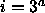CSE 5311 Section 501 Fall 1998
Homework 4
Due: March 25, 1998, in class

1. A sequence of n operations is performed on a data structure. The ith operation costs i if i is a power of 3 (i.e.,where a is a nonnegative integer); otherwise, the cost is 1.

• Use an aggregate analysis to determine the amortized cost per operation.
• Use a potential analysis to determine the amortized cost per operation.
• Use an accounting analysis to determine the amortized cost per operation.
2. Show the binomial heap that results after each operation listed below:

• Insert the following numbers, in order, into heap H1: 12, 7, 25, 15, 28, 33, 41 (show heap H1 after each step).
• Insert the following numbers, in order, into heap H2: 18, 3, 37, 6 (show heap H2 after each step).
• Merge heaps H1 and H2.
• Extract the minimum key from the merged heap.
3. Show the Fibonacci heap that results after each operation listed below:

• Insert the following numbers, in order, into heap H1: 12, 7, 25, 15, 28, 33, 41 (show heap H1 after the sequence).
• Insert the following numbers, in order, into heap H2: 18, 3, 37, 6 (show heap H2 after the sequence).
• Merge heaps H1 and H2.
• Extract the minimum key from the merged heap.
4. Implement code for inserting and deleting integer keys into a B-tree with minimum degree t=3 following the algorithms in chapter 19 of the textbook. You may assume that the entire tree resides in memory, so calls to Disk-Read and Disk-Write are unnecessary. The details are given below.

• Implement B-TREE-CREATE(T), B-TREE-SPLIT-CHILD(x,i,y), B-TREE-INSERT(T,k) and B-TREE-INSERT-NONFULL(x,k) according to the pseudocode in section 19.2 of the textbook.
• Implement B-TREE-DELETE(T,k) as described in section 19.3 of the textbook. Be sure to find the predecessor (2a) or successor (2b) and delete it in a single downward pass.
• Implement a clear manner of printing the tree structure and contents.

Once you have successfully compiled and executed your program on omega, send the code to cs5311-turnin@cse. The source code must be submitted by 5:30 p.m. on the due date. In addition to identifying yourself and the homework number in a comment at the top of your code, also include a ``Compile Using'' comment line so we know how to compile your program. Be sure to use good programming and documentation style.